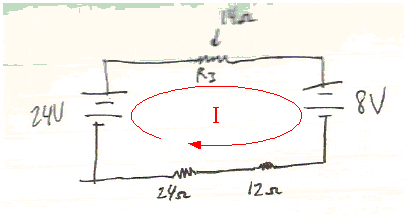Circuit help? with two batteries and resistors

Homework Statement

If R3 = 14Ω:
a. What is the current out of the 24 V battery?
b. What is the voltage drop across the R3 resistor?
c. What is the power dissipated by the 12 Ω resistor?

I=V/R
V=V(R3/Rtotal)
P=I2R

The Attempt at a Solution

Answers attached but I was unsure which side I should be using or if I am able to add up Voltage from batteries?
I worked it through, just need some clarification and was wondering if I followed through correctly!
Thanks

Attachments

• phys2.pdf
217.4 KB · Views: 1,422

gneill
Mentor
The circuit is connected in series, so the same current flows through all of the components. That means you need to take all the resistances and voltage supplies into account when calculating the current.One convenient way to write a suitable equation for finding I is to "walk" around the loop taking note of all potential rises and falls as you go. Potential rises or drops when you "walk over" a voltage supply depending upon the direction you're traveling; if you proceed from - to + as you go over it, it's a potential rise. For resistors, if you travel in the same direction as the current then there is a potential drop equal to I*R. The sum of all the potential rises and drops around a complete loop must be zero.

If you start at the bottom of the 24V supply and walk around the loop following the current as shown, can you write the sum of the rises and falls of potential?

Attachments

So does that mean the second battery actually decreases the voltage at 8V? or do the lines within the battery not switch to show direction?
I=(V1-V2)/(R+R+R) so
I=(24-8)/50=>8/25 or .32Amps
Vdrop=(.32)(14)=4.48V then another 8V from other battery. So before the last two resistors, 11.52V remain
V=(.32)(12)=3.84V
V=(.32)(24)=7.68V so after those last two resistors, V=0? does that seem right?

gneill
Mentor
So does that mean the second battery actually decreases the voltage at 8V? or do the lines within the battery not switch to show direction?
Right. When you pass through the 8V battery in the direction of the assumed current I, there is a voltage drop of 8V.
I=(V1-V2)/(R+R+R) so
I=(24-8)/50=>8/25 or .32Amps
Vdrop=(.32)(14)=4.48V then another 8V from other battery. So before the last two resistors, 11.52V remain
V=(.32)(12)=3.84V
V=(.32)(24)=7.68V so after those last two resistors, V=0? does that seem right?

Your result is okay, although you didn't have to calculate the current right away; you could have written out the equation for the entire loop first, then solved for I:

24V - I*14Ω - 8V - I*12Ω - I*24Ω = 0

Thus: I = (24V - 8V)/(14 + 12 + 24)Ω = 16V/50Ω = 0.32A

thank you!
then for b, voltage drop=.32(14)
and c, P=.322(12)=1.2288J?

gneill
Mentor
thank you!
then for b, voltage drop=.32(14)
and c, P=.322(12)=1.2288J?

That's the way to do itI appreciate the help!
Thanks for your time